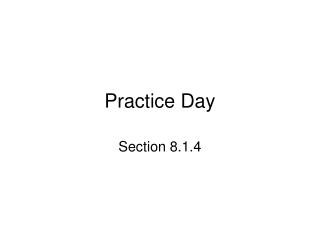DownloadDownload PresentationPractice Day

# Practice Day

Download Presentation## Practice Day

- - - - - - - - - - - - - - - - - - - - - - - - - - - E N D - - - - - - - - - - - - - - - - - - - - - - - - - - -
##### Presentation Transcript

1. Practice Day Section 8.1.4

2. Starter 8.1.4 • A manufacturer produces a large number of toasters. From past experience, he knows that about 2% are defective. In a quality control procedure, we randomly select 20 toasters for testing. We want to determine the probability that no more than one of these toasters is defective. • Is this a binomial setting? Justify your answer. • Find the probability that exactly one toaster is defective. • Find the probability that at most one toaster is defective. • Find the mean and standard deviation for the problem.

3. Objectives • Practice calculation with binomial distributions California Standards 5.0 Students know the definition of the mean of a discrete random variable and can determine the mean for a particular discrete random variable. 6.0 Students know the definition of the variance of a discrete random variable and can determine the variance for a particular discrete random variable. 7.0 Students demonstrate an understanding of the standard distributions (normal, binomial, and exponential) and can use the distributions to solve for events in problems in which the distribution belongs to those families.

4. Activity • Read pages 432 – 433 • Do problems 19 – 23